# CNN on FRDM_K64

cancel
Showing results for
Did you mean:

No ratings

CNN on FRDM_K64

# 1 Introduction

Limited by resources, ordinary MCU is difficult to do some complex deep learning. However, although it is difficult, it can still be done. CNN, convolutional neural network, is a kind of deep learning algorithm, which can be used to solve the classification task.

After the implementation of CNN, ordinary MCU can also be used as edge computing device. Next, we introduce how to run CNN on frdm-k64 to recognize handwritten numbers. The size of digital image is 28x28. 28x28 image as input for CNN will output a 1x10 matrix. There are few deep learning libraries written for MCU on the Internet. Even if there are, there will be various problems. NNoM framework is easy to transplant and apply, so we use it

# 2 Experiment

2.1 Required tools: frdm-k64, python 3.7, Pip, IAR, tcp232

https://github.com/majianjia/nnom This is a pure C framework that does not

rely on hardware structure. Transplantation is very convenient

2.3  we select the example ‘bubble’ to add the Inc, port and Src folders in NNoM to the project, as shown in the figure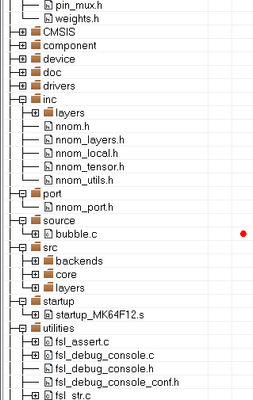Figure 1

Open the file ‘port.h’ . The definitation of NNOM_LOG is changed to PRINTF (__ VA_ ARGS__ , Open the ICF file, and change the heap size to 0x5000, define symbol__ size_ heap__ = 0x5000;

Malloc, which is used in this library, allocates memory from here. If it is small, it can't run the network

2.4 From the download framework, go into ‘mnist-simple/mcu’, which has trained file ‘weights.h’, and randomly generated handwritten image file, ‘image.h’. Add these two files to the project

#include "nnom_port.h"

#include "nnom.h"

#include "weights.h"

#include "image.h"

2.6 Delete the original code, add the following code

nnom_model_t *model;

const char codeLib[] = "@B%8&WM#*oahkbdpqwmZO0QLCJUYXzcvunxrjft/\\|()1{}[]?-_+~<>i!lI;:,\"^`'.   ";

/*******************************************************************************

* Code

******************************************************************************/

void print_img(int8_t * buf)

{

for(int y = 0; y < 28; y++)

{

for (int x = 0; x < 28; x++)

{

int index =  69 / 127.0 * (127 - buf[y*28+x]);

if(index > 69) index =69;

if(index < 0) index = 0;

PRINTF("%c",codeLib[index]);

PRINTF("%c",codeLib[index]);

}

PRINTF("\r\n");

}

}

// Do simple test using image in "image.h" with model created previously.

void mnist(char num)

{

uint32_t predic_label;

float prob;

int32_t index = num;

PRINTF("\nprediction start.. \r\n");

// copy data and do prediction

memcpy(nnom_input_data, (int8_t*)&img[index], 784);

nnom_predict(model, &predic_label, &prob);

//print original image to console

print_img((int8_t*)&img[index]);

PRINTF("\r\nTruth label: %d\n", label[index]);

PRINTF("\r\nPredicted label: %d\n", predic_label);

PRINTF("\r\nProbability: %d%%\n", (int)(prob*100));

}

int main(void)

{

uint8_t ch;

/* Board pin, clock, debug console init */

BOARD_InitPins();

BOARD_BootClockRUN();

BOARD_InitDebugConsole();

/* Print a note to terminal */

model = nnom_model_create();

// dummy run

model_run(model);

PRINTF("\r\nwhich image to distinguish 0-9 \r\n");

for(uint8_t i=0; i<10; i++)

{

print_img((int8_t*)&img[i]);

}

while(1)

{

PRINTF("\r\nwhich image to distinguish 0-9 \r\n");

ch = GETCHAR();

if((ch >'9') || ch < '0')

{

continue;

}

PRINTF("\r\n");

mnist(ch-'0');

}

}

An error will be reported when compiling ‘weights.h’, due to lack of few parameters.

In layer , layer , layer , you need to add ‘division (1,1)’ after ‘stride (1,1)’. In this way, the compilation passes.

2.7 As a result, open the serial port software. At the beginning, the terminal will print out a variety of handwritten digital pictures, and then enter a number,

The corresponded picture will be recognized.Figure 2

When we input ‘8’, the recognition is the handwriting '9'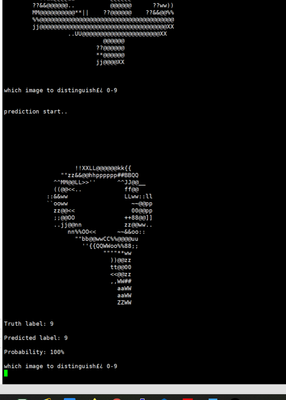Figure 3

The ‘Truth label’ corresponds to IMG9_LABLE in ‘image.h’ and ‘Predicted label’ is the prediction results

# 3 training

Through the above steps, we have realized a simple handwritten numeral recognition. Next, we will introduce ‘weights.h’. How to generate the weight model here? The image data here are all from MNIST digital set. How can we make a handwritten number for MCU to recognize?

3.1 Under ‘nnom-master\examples\mnist-simple’, there is a ‘mnist_ simple.py’. You need to run it to generate ‘weights.h’ and ‘image.h’. To run this, you need to install tensorflow, keras and so on. When you run it, you can use pip to install what is missing

The network operation process is as shown in the figure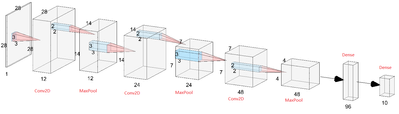Figure 4

Conv2d-> convolution operation, Maxpool-> pooling.

The meaning of convolution operation is to extract the features of the image. Pooling is a bit like compressing data, which can reduce the running space.

28x28 input and output a 1x10 matrix, representing the possibility of 0-9

3.2 We can use the ‘Paint’ program of WIN to adjust the canvas to 28x28, write numbers on it and save it in PNG format. I wrote a ‘4’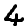Figure 5

Change the code as following.

nnom_model_t *model;

uint8_t temp[28*28]={0};

const char codeLib[] = "@B%8&WM#*oahkbdpqwmZO0QLCJUYXzcvunxrjft/\\|()1{}[]?-_+~<>i!lI;:,\"^`'.   ";

/*******************************************************************************

* Code

******************************************************************************/

void print_img(int8_t * buf)

{

for(int y = 0; y < 28; y++)

{

for (int x = 0; x < 28; x++)

{

int index =  69 / 127.0 * (127 - buf[y*28+x]);

if(index > 69) index =69;

if(index < 0) index = 0;

PRINTF("%c",codeLib[index]);

PRINTF("%c",codeLib[index]);

}

PRINTF("\r\n");

}

}

void mnist_pic(uint8_t *temp)

{

float prob;

uint32_t predic_label;

PRINTF("\nprediction start.. \r\n");

// copy data and do prediction

memcpy(nnom_input_data, (int8_t*)temp, 784);

nnom_predict(model, &predic_label, &prob);

//print original image to console

print_img((int8_t *)temp);

PRINTF("\r\nPredicted label: %d\n", predic_label);

PRINTF("\r\nProbability: %d%%\n", (int)(prob*100));

}

int main(void)

{

/* Board pin, clock, debug console init */

BOARD_InitPins();

BOARD_BootClockRUN();

BOARD_InitDebugConsole();

/* Print a note to terminal */

model = nnom_model_create();

// dummy run

model_run(model);

while(1)

{

PRINTF("\r\n Send picture by serial\r\n");

PRINTF("\r\n Got picture\r\n");

mnist_pic(temp);

}

}

3.3 Then use pic2mnist.py(see the attachment), run this script with CMD and

enter 'Python pic2mnist.py 1. PNG ', 1. PNG is the image to be parsed, and then ‘content.txt’ will be generated. The file contains the data of the picture. Send the data to the MCU through the serial port. Note that ‘Send as Hex’ should be checked. Similarly, the handwritten picture will be displayed first, and then the picture will be recognized.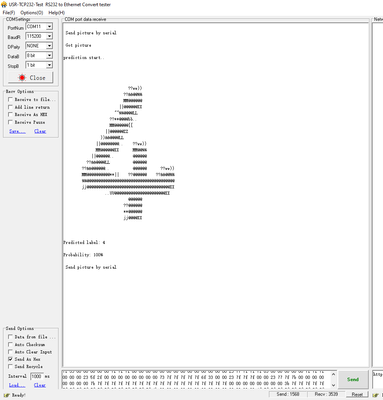Figure 6

We can see that '4' was identified

Labels (2)

• ### Kinetis K Series MCUs

Version history
Revision #:
2 of 2
Last update:
‎01-18-2021 07:25 PM
Updated by: